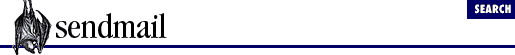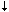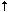home | O'Reilly's CD bookshelfs | FreeBSD | Linux | Cisco | Cisco Exam

#Chapter 31Defined Macros## 31.6 Macro Conditionals: \$?, \$|, and \$.

Occasionally, it is necessary to test a macro to see whether a value has been assigned to it. To perform such a test, a special prefix and two operators are used. The general form is

```
if

else

endif\$?x text1 \$| text2 \$.if
x
is defined   if
x
is not defined
```

This expression yields one of two possible values: ``` text1``` if the macro named ``` x``` has a value, ``` text2``` if it doesn't. The entire above expression, starting with the ``` \$?``` and ending with the ``` \$.``` , yields a single value, which may contain multiple tokens.

The following, for example, includes the configuration-file version in the SMTP greeting message but does so only if that version (in ``` \$v``` ; see Section 31.10.38 ) is defined:

```O SmtpGreetingMessage=\$j Sendmail (\$v/```

\$?Z\$Z\$|generic\$.
```note
```

Here the parenthetical version information is expressed one way if ``` Z``` has a value (like ``` 1.4``` ):

`(\$v/\$Z)`

but is expressed differently if ``` Z``` lacks a value:

`(\$v/generic)`

The else part (``` \$|``` ) of this conditional expression is optional. If it is omitted, the result is the same as if the ``` text2``` were omitted:

```\$?xtext1\$|\$.
\$?xtext1\$.```

Both of the preceding yield the same result. If ``` x``` has a value, then ``` text1``` becomes the value of the entire expression. If ``` x``` lacks a value, then the entire expression lacks a value (produces no tokens).

Note that it is not advisable to use the ``` \$?``` conditional expression in rules. It may not have the intended effect, because macro conditionals are expanded when the configuration file is read.

### 31.6.1 Conditionals May Nest

V8 sendmail allows conditionals to nest. To illustrate, consider the following expression:

`\$?x \$?y both \$| xonly \$. \$| \$?y yonly \$| none \$. \$.`

This is just like the example in the previous section:

`\$?x text1 \$| text2 \$.`

except that ``` text1``` and ``` text2``` are both conditionals:

```text1 = \$?y both \$| xonly \$.
text2 = \$?y yonly \$| none \$.```

The grouping when conditionals nest is from the outside in. In the following example, parentheses have been inserted to show the groupings (they are not a part of either expression):

``````

(
```
\$?x ```

(
```
text1```

)
```
\$| ```

(
```
text2```

)
```
\$.```

)
```

```

(
```
\$?x ```

(
```
\$?y both \$| xonly \$.```

)
```
\$| ```

(
```
\$?y yonly \$| none \$.```

)
```
\$.```

)
```
```

Interpretation is from left to right. The logic of the second line above is therefore this: If both ``` \$x``` and ``` \$y``` have values, the result is ``` both``` . If ``` \$x``` has a value but ``` \$y``` lacks one, the result is ``` xonly``` . If ``` \$x``` lacks a value but ``` \$y``` has one, the result is ``` yonly``` . And if both lack values, the result is ``` none``` .

The sendmail program does not enforce or check for balance in nested conditionals. Each ``` \$?``` should have a corresponding ``` \$.``` to balance it. If they do not balance, sendmail will not detect the problem. Instead, it may interpret the expression in a way that you did not intend.

The depth to which conditionals may be nested is limited only by our ability to easily comprehend the result. More than two deep is not recommended, and more than three deep is vigorously discouraged.31.5 Macro Expansion: \$ and \$&31.7 Categories of Macros